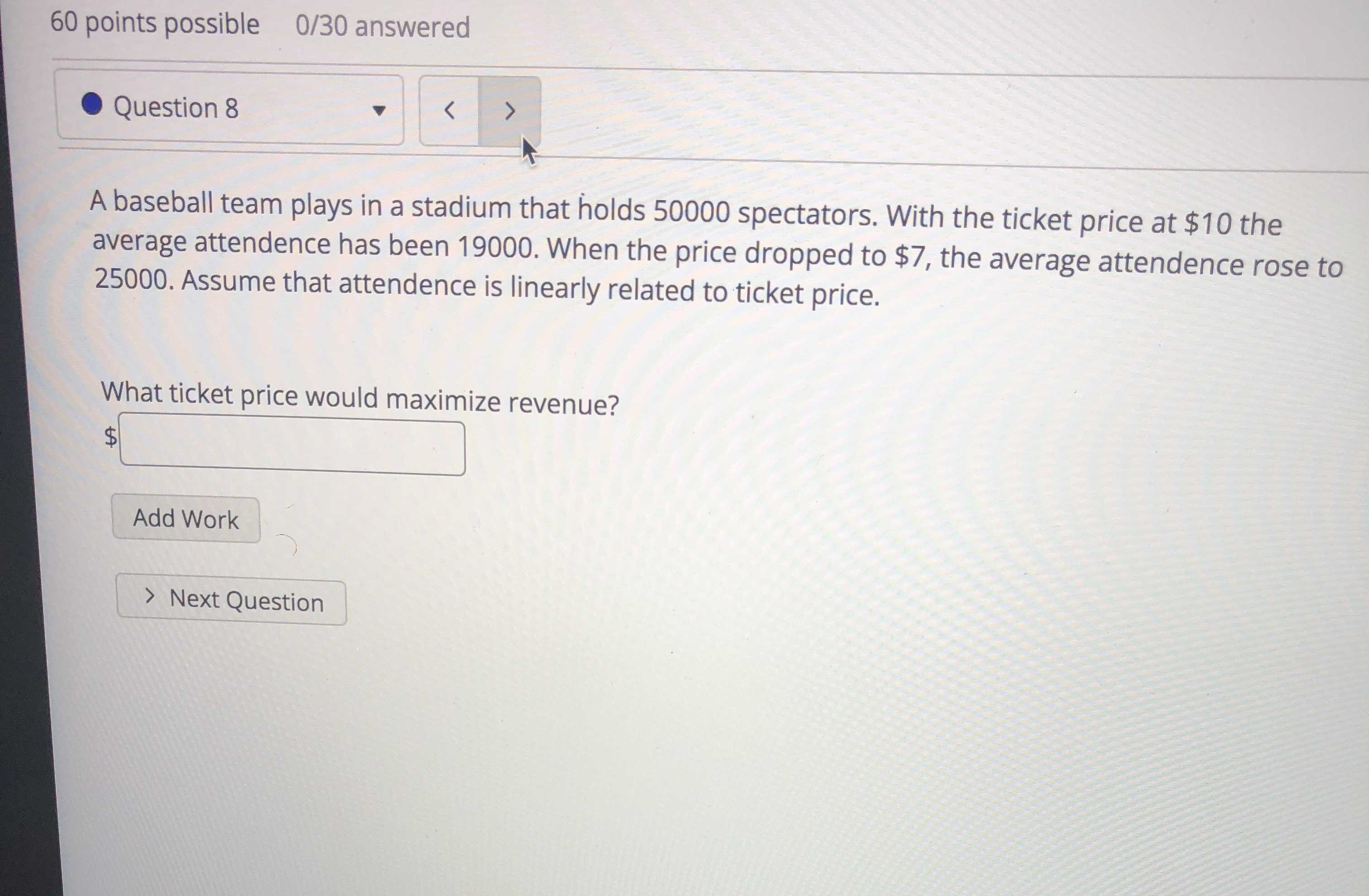### ¿Todavía tienes preguntas de matemáticas?

Pregunte a nuestros tutores expertos
Algebra
PreguntaA baseball team plays in a stadium that holds $$50000$$ spectators. With the ticket price at $$\ 10$$ the average attendance has been $$19000$$ . When the price dropped to $$\ 7 ,$$ the average attendance rose to $$25000 .$$ Assume that attendance is linearly related to ticket price.

What ticket price would maximize revenue?  \$$$\square$$